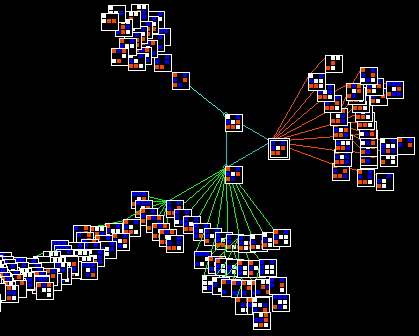part of a basin of attraction of a value v=4, neighborhood k=3, size n=9, 1d cellular automaton, with the nodes shown in 2d. The rule and other info is shown below. Below that, the color scheme for cell values.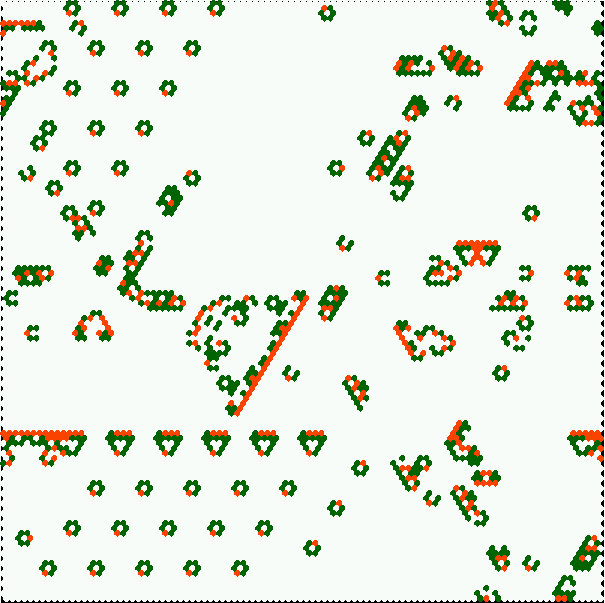complex kcode with many emerging gliders, glider guns, and self-replicating structures, on hex lattice. Click to enlarge, click here for info.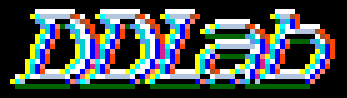Discrete Dynamics Lab

## Update Dec 2003

### DDLabVersion m04: multi value logic, totalistic rules, many new features

This is a major update. The two most significant changes:

• Generalizing all aspects of DDLab for multi-value logic (up to 8 values or colors), insead of just Boolean logic (0,1).
• Constraining DDLab to run forward only for two types of totalistic rules, which allows larger neighborhoods (up to k=25). In 2d the neighborhoods are predefined to make both square or hexagonal lattices. Many interesting "life"-like and other complex dynamics can be found in this constrained rule-space in 2d and 3d.
Click here for examples, details and instructions for running these complex rules.

Multi-value new DDLab includes many other new features, improvements and revisions, both major and minor, since the last official release in May 2002. Some of these, but not all, are listed below. The usual harvest of bugs have also been fixed (and not too may added - we hope!).

• Code: written in c, is available on request subject to some conditions.
• Manual: The DDLab manual released in July 2001 (that corresponds to DDLab Version 24) needs to be updated - we're working on it. Most explanations in it can be reinterpreted for the multi-value version by the astute reader. In the meantime this web page can serve as a temporary reference for some of the changes.

For previous updates since March 96,
click July 01, Feb 99, Sept 97.

### Forward only for just totalistic rules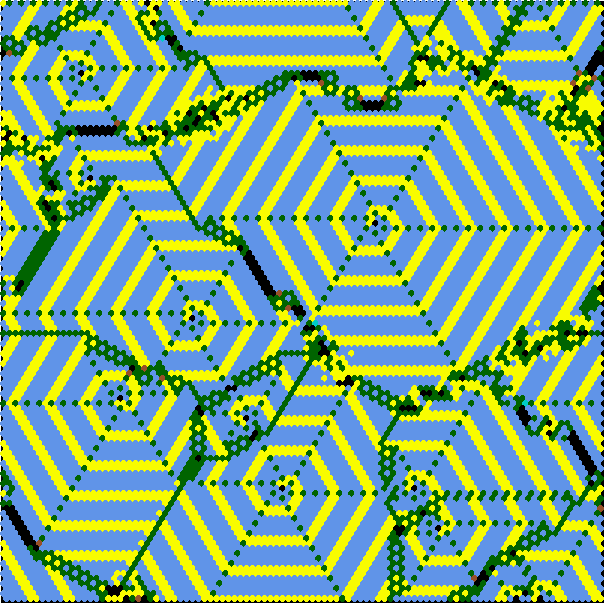complex kcode with emerging spirals on hex lattice. click to enlarge, click here for info
• This constrains DDLab:
• to run forward only, to generate and analyze space-time patterns, and disable all basin of attraction functions.
• to allow just t-totalistic (tcode) and k-totalistic rules (kcode).
The "life"-like rules belong to "kcode". (click here for an examples, explanations, instructions).
•  These constraints conserve memory by reducing the size of lookup tables, which allow bigger value-range/neighborhood (v/k) combinations. The max v/k supported is shown on the right. ``` v k k-totalistic table size 2 25 26 3 25 351 4 25 3276 5 25 23751 6 17 26334 7 13 27132 8 11 31824 ```
• The first prompt in DDLab's main sequence is now...
• Exit-q, graphics setup, randseed, totalistic/forward only-t
• forward only/single basin/subtree-s (def-field):

• Enter t to select forward only for just totalistic rules, the prompt changes to...
• Exit-q, graphics setup, randseed, allow basins-b
• totalistic rules and forward only (def):

### Multi-value logic

• The multi-value version of DDLab allows network elements to have a value range v anywhere from 2 to 8 (see preview). All DDLab functions (those described in the manual plus some new functions) work for multi-value, including setting rules and initial states, attractor basins including compression, in-degree histograms, learning/forgetting, space-time patterns, filtering, input-entropy, automatic classification, samples of glider rules, the reverse algorithms for CA, RBN and random maps, the Z and lambda parameters, the meta-graph, canalizing, the Derrida plot, damage spread, etc. For 2 values, DDLab works much as before, but with various additional functions as will be described.

• The second prompt in DDLab's main sequence is now...
Value range (def 2, max 8):
Enter the value range (or return for the default, which changes following previous selections).

• There is a color scheme for cell values, displayed top right or left at various times.

• The neighborhood matrix is generalized for multi-value (and can be rescaled).v4k3 neighborhood matrix, sets out all possible neighborhoods (vertically) in descending order of their 4-ary decimal equivalents. The rule-table itself assigns a output value for each neighborhood.

•  Rule tables can become very long with bigger value-range/neighborhood (v/k). The max v/k supported for full lookup tables (which allow basins of attraction) is shown on the right. ``` v k k-totalistic table size 2 13 8162 3 9 19683 4 7 16484 5 6 15629 6 5 7776 7 5 16807 8 4 4096 ```
•  State-space S also increases rapidly with the value-range v, and network size n (S=vn). To generate the basin of attraction field (not single basins or subtrees) the max v/n supported is shown on the right. ``` v n 2 31 3 20 4 16 5 13 6 12 7 11 8 10 ```

### 2d neighborhoods (square and hex) updated

As mentioned earlier, the max neighborhood size when DDLab is constrained to run forward only, for just totalistic rules, is k=25 (for v>5 k is reduced). For the full lookup table max k=13 (for v>3 k is also reduced).

The predefined 2d neighborhoods have been extended up to k=25. Some k have a layout for both a square and hex lattice. Others have just hex or square for best symmetry. In the case of both, square is the default. To force hex where its available there is a new option in the top right WIRING prompt.

• 2d-2 (hex-x) Enter 2x to force a hex lattice if available.
Below are all predefined neighborhoods (defaults shown with a black background).
Note that the center cell may form part of its neighborhood (or not). If it does it is shown in red.
 k=4 k=5 k=6 k=7 k=8 k=9 k=10 k=11 k=12 k=13 k=14 k=15 k=16 k=17 k=18 k=19 k=20 k=21 k=22 k=23 k=24 k=25 squarelattice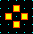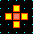none none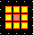none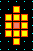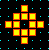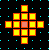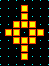none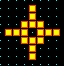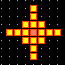none none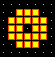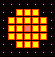none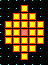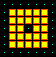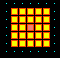hexlattice none none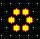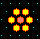none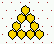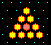none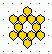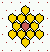none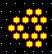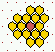none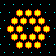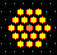none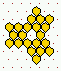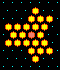none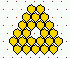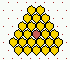### Toggling between square and hex presentation

In the 2d wiring graphic prompts, there is a new prompt,
• CA wiring and tog hex-x
This toggles the lattice presentation between hex and square, changes the highlighted cell's neighborhood to a local CA predefined neighborhood, and toggles the neighborhood between hex and square (if possible).

In the "on the fly" prompts when running forward in 2d, there is a new prompt to change between square or hex presentation,

• tog hex (on) ...present status is indicated by (on)/(of)
For CA with a square neighborhood, the 2d space-time pattern will start on a square lattice, if hex on a hex lattice.

### Setting rules/states updated

• The methods for setting rules and initial states (which are very similar) have been updated for multi-value, and some new methods added. For example...Creating an initial state with the bit drawing options for a 2d network, v=8.

• fill-f gives a further prompt for the value (color) to be filled.
• bits-b drawing bits with the mouse or keyboard, the value (color) can be selected.
• printxterm-x to show the lookup table in the xterm window, after setting the rule, backtrack to the rule selection prompt, then enter x.
• in the bits-b drawing options,
• width-w a new option , allows the line width to be increased from 1 to many cells for a broader brush stroke.
• toghex-x the 2d layout can be toggled between square and hexagonal.
• file-F to save patterns or selected patches, or to load patterns, or patches in a selected position.

This is the updated top right prompt with the various bit drawing options if v=4...

• keys: set/select/draw value 3-0, vert-v
• mouse: move-click, draw selected-keep pressed, move-arrows
• buttons: 1-draw 0-comp width-w, pan2d up/down-U/D exp/cont-e/c
• rotate-l/r/u/d, comp-m, toghex-x file-F accept-ret

### Classification of rule-space and complex rule sample

The automatic classification of rule space has been updated for multi-value. Samples of glider/complex rules are available that can be randomly set on-the-fly when running forward, with g,
 These files are in dd_extra.tar.gz and include the following, rule sample automatic classification files: for 1d, v=2, full lookup table, as before but renamed, v2k5ss.sta (k=5) v2k6ss.sta (k=6) v2k6ss.sta (k=7) for 1d, multi_value, full lookup table, v3k3ss.sta (v=3 k=3) v4k2ss.sta (v=4 k=2) v4k3ss.sta (v=4 k=3) v5k2ss.sta (v=5 k=2) for 2d, multi-valye kcode, v3k4bs.sta (v=3 k=4) v3k5bs.sta (v=3 k=5) v3k6bs.sta (v=3 k=6) v3k7bs.sta (v=3 k=7) v4k4bs.sta (v=4 k=4) v4k6bs.sta (v=4 k=6) glider/complex rule sample files for loaded on-the-fly with "g": for 1d, v=2, full lookup table, as before but renamed, g_v2k5.r_s (k=5) g_v2k6.r_s (k=6) g_v2k7.r_s (k=7) for 1d, multi_value, full lookup table, g_v3k3.r_s (v=3 k=3) g_v3k4.r_s (v=3 k=4) g_v4k2.r_s (v=4 k=2) for 2d/3d, kcode lookup table, g_v3k6.r_v (v=3 k=6)An automatically classified sample of about 16000 v=3 k=6 kcode rules for 2d hex CA Axies are as follows: x=standard deviation of the input-entropy, y=mean entropy, z=frequency of rules on the xy scatter plot. Complex rules are spread out on the right with higher standard deviation, Chaotic and ordered rules are on the left with low standard deviation - chaos has high entropy (the tower), and order lower entropy.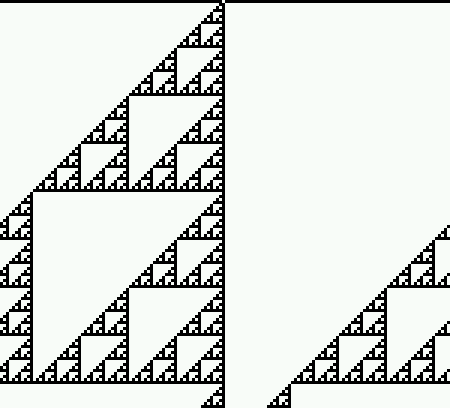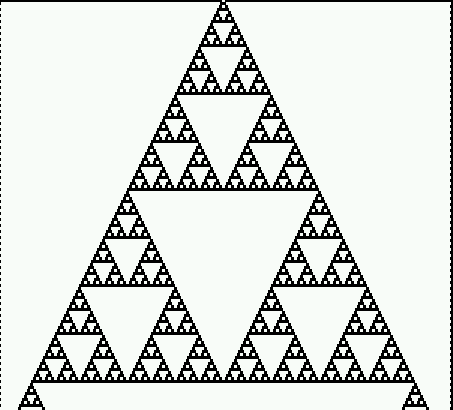The "un-slant" option, rule 153, Click to enlarge.

### New/revised on-the-fly options

When running forward, there are some new and revised on-the-fly options as follows:

change seed size

• 4/v/o..rnd seed/block/orig
• 4 resets the pattern, the density predefined.
• v sets a random block, the size and density predefined.
• o restores the original initial state.
• 5/6..singleton pos/neg for multi-value both set a cell with a random color against a
background of a different random color. For v=2 (as before) 5 sets a positive seed
(a central 1 with a background of 0s), 6 sets a negative seed.
• l/L..rnd value/block
• l randomly flips the value of a cell at a random position.
• L adds a random central block, where the size of the block was defined before.
presentation
• x..tog slant (off) for 1d, some CA space-time patterns are asymmetric, for example rule 60.
This option makes the space-time patterns appear symmetric.
• x..tog hex (off) for 2d, toggle the 2d lattice between square and hex (discused above).
• In both cases the present status is indicated by (on)/(of).
• 0..rearrange cell color randomly rearranges the allocation of colors to values.

### Falling of edge boundary conditions

By default, the boundary condition in DDLab are periodic, a ring for 1d, a torus for 2d and a 3d torus for 3d. There is a new option, in the top right space-time pattern interrupt options, to set a fixed border of a given value and width.
• fixed border-B If B is selected, there is a further prompt,
• fixed border: width (0-25, now 0):
• border value: (0-2, now 0):
If a suitable border width, and border value (same as the background), are set, gliders and other structures in 2d and 3d complex CA can seem to fall of the edge, simulating an infinite space, though structures sometimes interact with the edge.